Next: Method for Occlusions Breaking Up: Occlusion Understanding Previous: Occlusion Understanding

## Method for Occlusions Preserving Surfaces and Occlusions Breaking Surfaces [1, 6]

Image Segmentation
The image range can be segmented by extracting depth discontinuities either across each coordinate axis , or between neighbouring fitted 3D surfaces . The case of depth discontinuities is represented in figure 3, while the case of 3D surfaces is represented in figure 4.

The extracted surfaces are further segmented by analysing their surface orientation. This process is called fold edge detection. The result of this detection is shown in figure 5. The right-hand figure shows that a fold edge detection leads to a more accurate detection.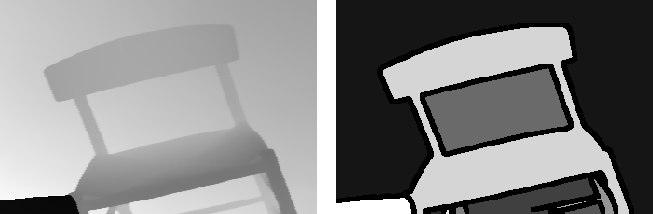Figure 3: A Range image (left) and corresponding extracted regions after labelling (right).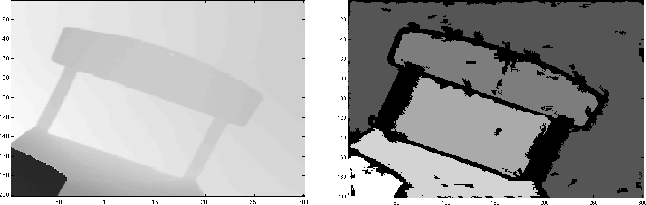Figure 4: The first segmentation.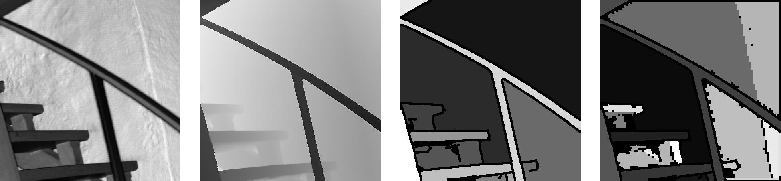Figure 5: An intensity image (left) and its corresponding range image (center-left). The same image after depth discontinuity processing (center-right) and additional partitioned using fold edges (right).

Surface Fitting
The extracted surfaces are then fitted by parametric surface shapes in order to obtain good comparative measures. Experiments have shown that planes, cylinders and spheres are usually sufficient to describe most of the simple surfaces in architectural scenes. The following table shows the parameters associated with each surface type.

 Surface type Description Planar surface normaldisplacement disp Cylindrical point on axis p (circular) unit vector of axis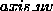radius r Spherical centre c radius r

For two surfaces to be contiguous, they must be first of the same type. The surface parameters introduced above can be used for comparison. The following table gives the parameters and the thresholds used for determining whether two surfaces match or not.

 Surface type Requirements for matching Plane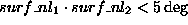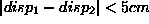Cylinder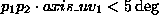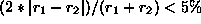Sphere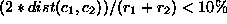Next: Method for Occlusions Breaking Up: Occlusion Understanding Previous: Occlusion Understanding

Bob Fisher
Thu Jan 17 16:45:14 GMT 2002